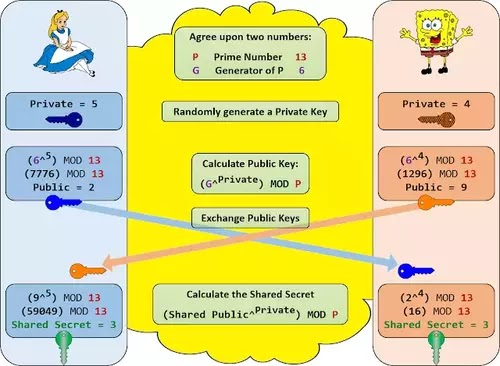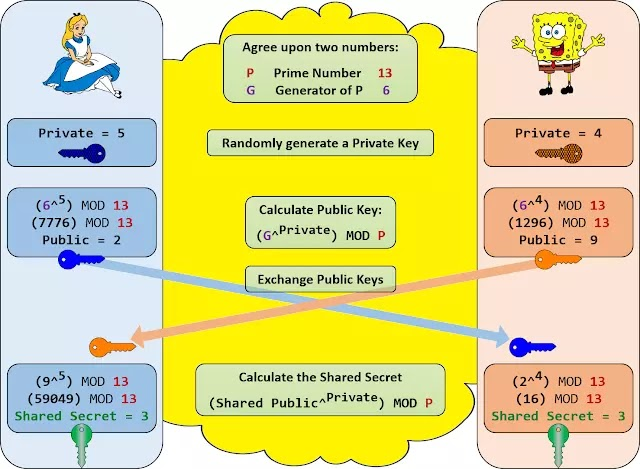# Diffie Hellman Key exchange algorithm Implementation in C## Diffie Hellman Key exchange algorithm:

The Diffie-Hellman key exchange algorithm is a method for securely exchanging keys over a public communication channel. It allows two parties to agree on a shared secret key without any prior secret information shared between them.Diffie Hellman Key exchange algorithm Implementation in C

It is primarily used to exchange cryptography keys for symmetric encryption algorithms. It was proposed in 1976 by Whitfield Diffie and Martin Hellman. Diffie-Hellman is currently used in many protocols like Secure Sockets Layer (SSL)/Transport Layer Security (TLS), Secure Shell (SSH), Internet Protocol Security (IPSec), and Public Key Infrastructure (PKI).

### Steps of Diffie Hellman key exchange Algorithm:

1       Requires two large numbers, one prime (P) and (G), a primitive root of P

2       P and G are both publicly available numbers

a.       P is at least 512 bits

3       Users pick private values a and b

4       Compute public values

a.       x = ga mod p

b.      y = GB mod p

5       Public values x and y are exchanged

6       Compute shared, private key

a.       ka = yamod p

b.      kb = xbmod p

c.       Algebraically, it can be shown that ka = kb

Users now have a symmetric secret key to encrypt.

### Example of Diffie Hellman Key exchange algorithm Implementation

1. Alice and Bob get public numbers
1. P = 23, G = 9
2. Alice and Bob compute public values
3. X = 94 mod 23 = 6561 mod 23 = 6
4. Y = 93 mod 23 = 729 mod 23    = 16
5. Alice and Bob exchange public numbers
2. Alice and Bob compute symmetric keys
1. ka = ya mod p = 164 mod 23 = 9
2. kb = xb mod p = 63 mod 23 = 9

3.       9 is the shared secret.

### The output of the Diffie Hellman Key exchange algorithm Implementation in C:

 Diffie Hellman Key exchange algorithm Implementation in C

### Summary:

The Diffie-Hellman key exchange algorithm is a secure method for exchanging keys over a public communication channel. It enables two parties to agree on a shared secret key without any prior confidential information shared between them. The algorithm is widely used in many protocols like SSL/TLS, SSH, IPSec, and PKI. Its implementation involves the use of large prime numbers and primitive roots and the computation of public and private values that are exchanged between the parties to generate a shared secret key. The algorithm's security is based on the difficulty of computing discrete logarithms.

#### RSA Algorithm(Encryption and Decryption) implementation in C

1.1.